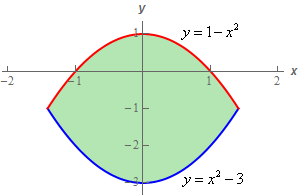Paul's Online Notes
Home / Calculus III / Multiple Integrals / Double Integrals over General Regions
Show Mobile Notice Show All Notes Hide All Notes
Mobile Notice
You appear to be on a device with a "narrow" screen width (i.e. you are probably on a mobile phone). Due to the nature of the mathematics on this site it is best views in landscape mode. If your device is not in landscape mode many of the equations will run off the side of your device (should be able to scroll to see them) and some of the menu items will be cut off due to the narrow screen width.

### Section 15.3 : Double Integrals over General Regions

12. Use a double integral to determine the area of the region bounded by $$y = 1 - {x^2}$$ and $$y = {x^2} - 3$$.

Show All Steps Hide All Steps

Start Solution

Okay, we know that the area of any region $$D$$ can be found by evaluating the following double integral.

$A = \iint\limits_{D}{{dA}}$

For this problem $$D$$ is the region sketched below.Show Step 2

We’ve done enough double integrals by this point that it should be pretty obvious the best order of integration is to integrate with respect to $$y$$ first.

Here are the limits for the integral with this order.

$\begin{array}{c} - \sqrt 2 \le x \le \sqrt 2 \\ {x^2} - 3 \le y \le 1 - {x^2}\end{array}$

The $$x$$ limits can easily be found by setting the two equations equal and solving for $$x$$.

Show Step 3

The integral for the area is then,

$A = \iint\limits_{D}{{dA}} = \int_{{ - \sqrt 2 }}^{{\sqrt 2 }}{{\int_{{{x^2} - 3}}^{{1 - {x^2}}}{{\,dy}}\,dx}}$ Show Step 4

Now all we need to do is evaluate the integral. Here is the $$y$$ integration.

$A = \iint\limits_{D}{{dA}} = \int_{{ - \sqrt 2 }}^{{\sqrt 2 }}{{\left. y \right|_{{x^3} - 3}^{1 - {x^2}}\,dx}} = \int_{{ - \sqrt 2 }}^{{\sqrt 2 }}{{4 - 2{x^2}\,dx}}$ Show Step 5

Finally, the $$x$$ integration and hence the area of $$D$$ is,

$A = \left. {\left( {4x - \frac{2}{3}{x^3}} \right)} \right|_{ - \sqrt 2 }^{\sqrt 2 } = \require{bbox} \bbox[2pt,border:1px solid black]{{\frac{{16\sqrt 2 }}{3}}}$